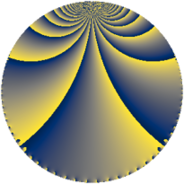# Properties

 Label 3136.2.b.bLevel $3136$ Weight $2$ Character orbit 3136.b Analytic conductor $25.041$ Analytic rank $0$ Dimension $2$ CM discriminant -8 Inner twists $4$

# Learn more

## Newspace parameters

 Level: $$N$$ $$=$$ $$3136 = 2^{6} \cdot 7^{2}$$ Weight: $$k$$ $$=$$ $$2$$ Character orbit: $$[\chi]$$ $$=$$ 3136.b (of order $$2$$, degree $$1$$, minimal)

## Newform invariants

 Self dual: no Analytic conductor: $$25.0410860739$$ Analytic rank: $$0$$ Dimension: $$2$$ Coefficient field: $$\Q(\sqrt{-1})$$ Defining polynomial: $$x^{2} + 1$$ Coefficient ring: $$\Z[a_1, a_2, a_3]$$ Coefficient ring index: $$2$$ Twist minimal: no (minimal twist has level 64) Sato-Tate group: $\mathrm{U}(1)[D_{2}]$

## $q$-expansion

Coefficients of the $$q$$-expansion are expressed in terms of $$i = \sqrt{-1}$$. We also show the integral $$q$$-expansion of the trace form.

 $$f(q)$$ $$=$$ $$q + 2 i q^{3} - q^{9} +O(q^{10})$$ $$q + 2 i q^{3} - q^{9} + 6 i q^{11} + 6 q^{17} + 2 i q^{19} + 5 q^{25} + 4 i q^{27} -12 q^{33} + 6 q^{41} -10 i q^{43} + 12 i q^{51} -4 q^{57} -6 i q^{59} + 14 i q^{67} -2 q^{73} + 10 i q^{75} -11 q^{81} + 18 i q^{83} -18 q^{89} -10 q^{97} -6 i q^{99} +O(q^{100})$$ $$\operatorname{Tr}(f)(q)$$ $$=$$ $$2q - 2q^{9} + O(q^{10})$$ $$2q - 2q^{9} + 12q^{17} + 10q^{25} - 24q^{33} + 12q^{41} - 8q^{57} - 4q^{73} - 22q^{81} - 36q^{89} - 20q^{97} + O(q^{100})$$

## Character values

We give the values of $$\chi$$ on generators for $$\left(\mathbb{Z}/3136\mathbb{Z}\right)^\times$$.

 $$n$$ $$197$$ $$1471$$ $$1473$$ $$\chi(n)$$ $$-1$$ $$1$$ $$1$$

## Embeddings

For each embedding $$\iota_m$$ of the coefficient field, the values $$\iota_m(a_n)$$ are shown below.

For more information on an embedded modular form you can click on its label.

Label $$\iota_m(\nu)$$ $$a_{2}$$ $$a_{3}$$ $$a_{4}$$ $$a_{5}$$ $$a_{6}$$ $$a_{7}$$ $$a_{8}$$ $$a_{9}$$ $$a_{10}$$
1569.1
 − 1.00000i 1.00000i
0 2.00000i 0 0 0 0 0 −1.00000 0
1569.2 0 2.00000i 0 0 0 0 0 −1.00000 0
 $$n$$: e.g. 2-40 or 990-1000 Significant digits: Format: Complex embeddings Normalized embeddings Satake parameters Satake angles

## Inner twists

Char Parity Ord Mult Type
1.a even 1 1 trivial
8.d odd 2 1 CM by $$\Q(\sqrt{-2})$$
4.b odd 2 1 inner
8.b even 2 1 inner

## Twists

By twisting character orbit
Char Parity Ord Mult Type Twist Min Dim
1.a even 1 1 trivial 3136.2.b.b 2
4.b odd 2 1 inner 3136.2.b.b 2
7.b odd 2 1 64.2.b.a 2
8.b even 2 1 inner 3136.2.b.b 2
8.d odd 2 1 CM 3136.2.b.b 2
21.c even 2 1 576.2.d.a 2
28.d even 2 1 64.2.b.a 2
35.c odd 2 1 1600.2.d.a 2
35.f even 4 1 1600.2.f.a 2
35.f even 4 1 1600.2.f.b 2
56.e even 2 1 64.2.b.a 2
56.h odd 2 1 64.2.b.a 2
84.h odd 2 1 576.2.d.a 2
112.j even 4 1 256.2.a.a 1
112.j even 4 1 256.2.a.d 1
112.l odd 4 1 256.2.a.a 1
112.l odd 4 1 256.2.a.d 1
140.c even 2 1 1600.2.d.a 2
140.j odd 4 1 1600.2.f.a 2
140.j odd 4 1 1600.2.f.b 2
168.e odd 2 1 576.2.d.a 2
168.i even 2 1 576.2.d.a 2
224.v odd 8 4 1024.2.e.l 4
224.x even 8 4 1024.2.e.l 4
280.c odd 2 1 1600.2.d.a 2
280.n even 2 1 1600.2.d.a 2
280.s even 4 1 1600.2.f.a 2
280.s even 4 1 1600.2.f.b 2
280.y odd 4 1 1600.2.f.a 2
280.y odd 4 1 1600.2.f.b 2
336.v odd 4 1 2304.2.a.h 1
336.v odd 4 1 2304.2.a.i 1
336.y even 4 1 2304.2.a.h 1
336.y even 4 1 2304.2.a.i 1
560.be even 4 1 6400.2.a.a 1
560.be even 4 1 6400.2.a.x 1
560.bf odd 4 1 6400.2.a.a 1
560.bf odd 4 1 6400.2.a.x 1

By twisted newform orbit
Twist Min Dim Char Parity Ord Mult Type
64.2.b.a 2 7.b odd 2 1
64.2.b.a 2 28.d even 2 1
64.2.b.a 2 56.e even 2 1
64.2.b.a 2 56.h odd 2 1
256.2.a.a 1 112.j even 4 1
256.2.a.a 1 112.l odd 4 1
256.2.a.d 1 112.j even 4 1
256.2.a.d 1 112.l odd 4 1
576.2.d.a 2 21.c even 2 1
576.2.d.a 2 84.h odd 2 1
576.2.d.a 2 168.e odd 2 1
576.2.d.a 2 168.i even 2 1
1024.2.e.l 4 224.v odd 8 4
1024.2.e.l 4 224.x even 8 4
1600.2.d.a 2 35.c odd 2 1
1600.2.d.a 2 140.c even 2 1
1600.2.d.a 2 280.c odd 2 1
1600.2.d.a 2 280.n even 2 1
1600.2.f.a 2 35.f even 4 1
1600.2.f.a 2 140.j odd 4 1
1600.2.f.a 2 280.s even 4 1
1600.2.f.a 2 280.y odd 4 1
1600.2.f.b 2 35.f even 4 1
1600.2.f.b 2 140.j odd 4 1
1600.2.f.b 2 280.s even 4 1
1600.2.f.b 2 280.y odd 4 1
2304.2.a.h 1 336.v odd 4 1
2304.2.a.h 1 336.y even 4 1
2304.2.a.i 1 336.v odd 4 1
2304.2.a.i 1 336.y even 4 1
3136.2.b.b 2 1.a even 1 1 trivial
3136.2.b.b 2 4.b odd 2 1 inner
3136.2.b.b 2 8.b even 2 1 inner
3136.2.b.b 2 8.d odd 2 1 CM
6400.2.a.a 1 560.be even 4 1
6400.2.a.a 1 560.bf odd 4 1
6400.2.a.x 1 560.be even 4 1
6400.2.a.x 1 560.bf odd 4 1

## Hecke kernels

This newform subspace can be constructed as the intersection of the kernels of the following linear operators acting on $$S_{2}^{\mathrm{new}}(3136, [\chi])$$:

 $$T_{3}^{2} + 4$$ $$T_{5}$$ $$T_{17} - 6$$ $$T_{31}$$

## Hecke characteristic polynomials

$p$ $F_p(T)$
$2$ $$T^{2}$$
$3$ $$4 + T^{2}$$
$5$ $$T^{2}$$
$7$ $$T^{2}$$
$11$ $$36 + T^{2}$$
$13$ $$T^{2}$$
$17$ $$( -6 + T )^{2}$$
$19$ $$4 + T^{2}$$
$23$ $$T^{2}$$
$29$ $$T^{2}$$
$31$ $$T^{2}$$
$37$ $$T^{2}$$
$41$ $$( -6 + T )^{2}$$
$43$ $$100 + T^{2}$$
$47$ $$T^{2}$$
$53$ $$T^{2}$$
$59$ $$36 + T^{2}$$
$61$ $$T^{2}$$
$67$ $$196 + T^{2}$$
$71$ $$T^{2}$$
$73$ $$( 2 + T )^{2}$$
$79$ $$T^{2}$$
$83$ $$324 + T^{2}$$
$89$ $$( 18 + T )^{2}$$
$97$ $$( 10 + T )^{2}$$
show more
show less